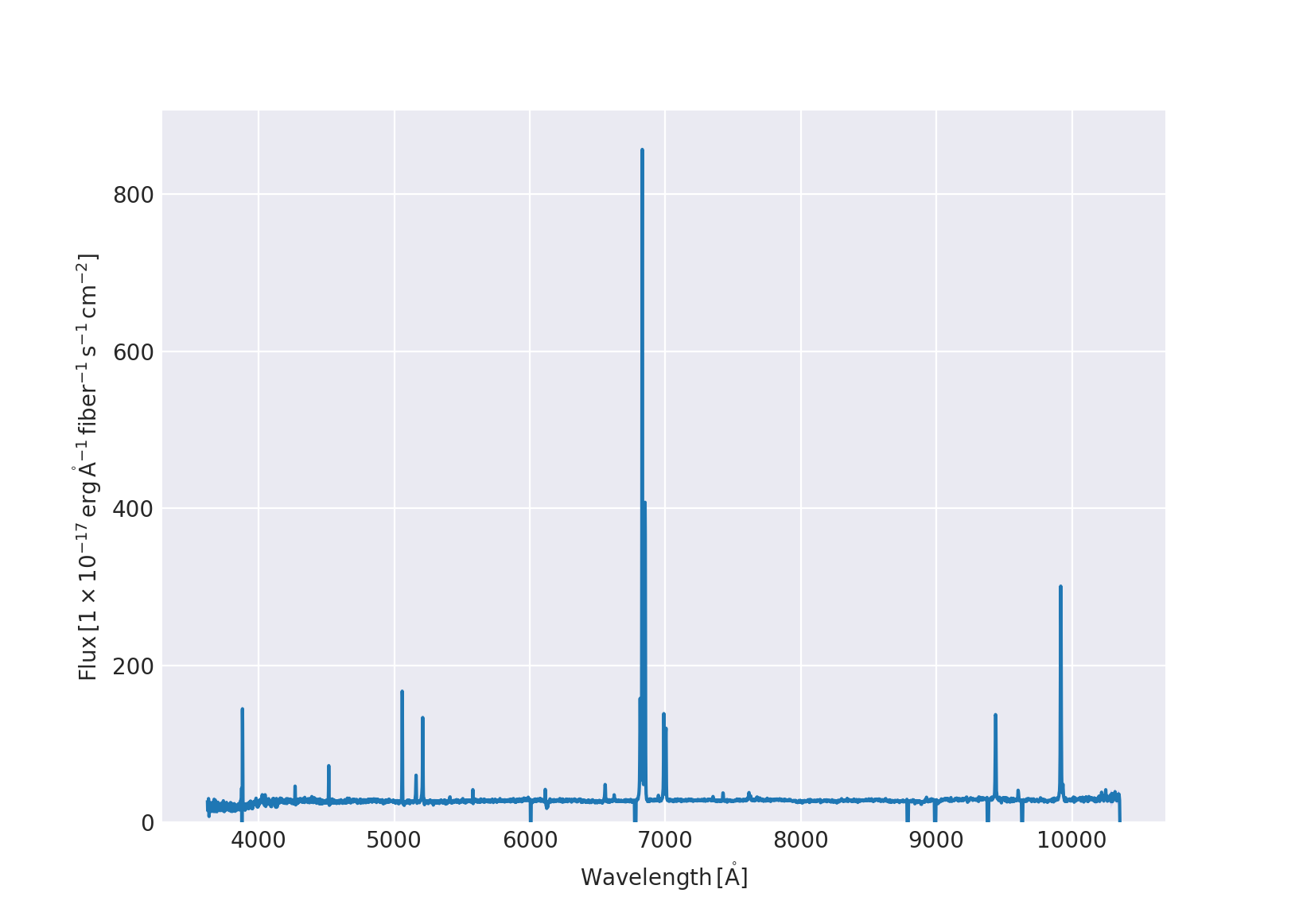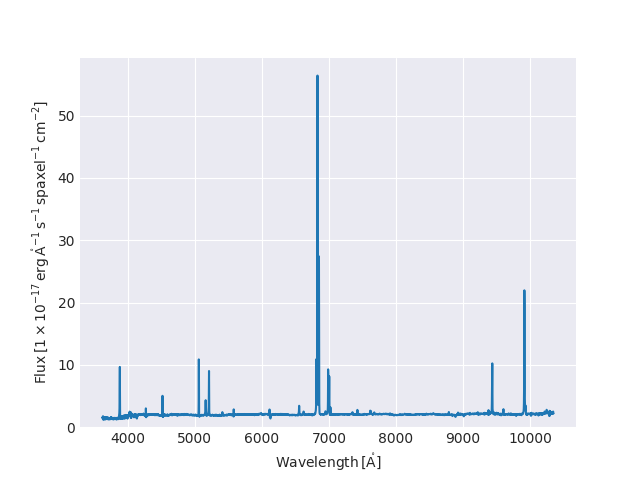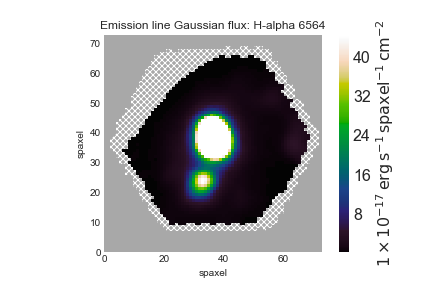# How to View Data with Marvin

Back to MaNGA tutorials

Here we show some simple examples of how to view MaNGA spectral data using Marvin, a new python module specifically to download, analyze, and visualize MaNGA data. If you're using Marvin for the first time, you'll want to follow the instructions to install the package and configure it to automatically download data.

from marvin.tools import RSS


The rss object is a list of fibers associated with the target galaxy, broken up by MJD, exposure number, or dither set. Let's access a single RSS fiber and plot it.

# grab the fiber closest to the center of the target galaxy, index 1313
fiber.plot()## Cube

To load a cube into Marvin, first load the Cube tool and then instantiate the cube

from marvin.tools.cube import Cube
cube = Cube(plateifu='7443-12703')


We can easily pull out a spectrum at any spaxel and plot it.

spaxel = cube.getSpaxel(0,0)
spaxel.flux.plot()Alternatively, you can pull out a spectrum using on-sky coordinates

spaxel=cube.getSpaxel(ra=229.525575871,dec=42.7458424664)


## Plot an Hα narrow band image

With the release of the Data Analysis Pipeline (DAP) products, several narrow-band images of key spectral features have already been created. First, we need to access the Maps for our objects:

maps = cube.getMaps()


There are two types of narrow-band images: one generated from Gaussian fits to the emission lines (the "emline_gflux" extension), and one that simply sums up the channels around each emission line (the "emline_sflux" extension). Let's plot the latter:

hamap = maps.getMap('emline_sflux',channel='ha_6564')
hamap.plot()